ARIMA and SARIMAX models with python

If you like  Skforecast ,  please give us a star on   GitHub! ⭐️

# ARIMA and SARIMAX models with Python

Joaquín Amat Rodrigo, Javier Escobar Ortiz
September, 2023## Introduction¶

ARIMA (AutoRegressive Integrated Moving Average) and SARIMAX (Seasonal AutoRegressive Integrated Moving Average with eXogenous regressors) are widely recognized and extensively utilized statistical forecasting models. This model comprises three components. The autoregressive element (AR) relates the current value to past (lagged) values. The moving average element (MA) assumes that the regression error is a linear combination of past forecast errors. Finally, the integrated component (I) indicates that the data values have been replaced with the difference between their values and the previous ones (and this differencing process may have been performed more than once).

While ARIMA models are well-known, SARIMAX models expand on the ARIMA framework by seamlessly incorporating seasonal patterns and exogenous variables.

In the ARIMA-SARIMAX model notation, the parameters $p$, $d$, and $q$ represent the autoregressive, differencing, and moving-average components, respectively. $P$, $D$, and $Q$ denote the same components for the seasonal part of the model, with $m$ representing the number of periods in each season.

• $p$ is the order (number of time lags) of the autoregressive part of the model.

• $d$ is the degree of differencing (the number of times that past values have been subtracted from the data).

• $q$ is the order of the moving average part of the model.

• $P$ is the order (number of time lags) of the seasonal part of the model.

• $D$ is the degree of differencing (the number of times the data have had past values subtracted) of the seasonal part of the model.

• $Q$ is the order of the moving average of the seasonal part of the model.

• $m$ refers to the number of periods in each season.

When the terms $P$, $D$, $Q$, and $m$ are zero and no exogenous variables are included in the model, the SARIMAX model is equivalent to an ARIMA.

Several Python libraries implement ARIMA-SARIMAX models. Four of them are:

• statsmodels: is one of the most complete libraries for statistical modeling in Python. Its API is often more intuitive for those coming from the R environment than for those used to the object-oriented API of scikit-learn.

• pmdarima: This is a wrapper for statsmodels SARIMAX. Its distinguishing feature is its seamless integration with the scikit-learn API, allowing users familiar with scikit-learn's conventions to seamlessly dive into time series modeling.

• skforecast: Among its many forecasting features, it has a new wrapper of statsmodels SARIMAX that also follows the scikit-learn API. This implementation is very similar to that of pmdarima, but has been simplified to include only the essential elements for skforecast, resulting in significant speed improvements.

• statsForecast: It offers a collection of widely used univariate time series forecasting models, including automatic ARIMA, ETS, CES, and Theta modeling optimized for high performance using numba.

This guide delves into three of these libraries - statsmodels, pmdarima, and skforecast - and explains how to building ARIMA-SARIMAX models using each. In addition, the introduction of the ForecasterSarimax class extends the capabilities of ARIMA-SARIMAX models by incorporating functionalities such as backtesting, hyperparameter tuning, probabilistic forecasting, and more.

Note

For a more detailed explanation of ARIMA-SARIMAX models visit:

## Libraries¶

Libraries used in this document.

In :
# Libraries
# ======================================================================================
import numpy as np
import pandas as pd
from io import StringIO
import contextlib
import re
import matplotlib.pyplot as plt
plt.style.use('seaborn-v0_8-darkgrid')

# pmdarima
from pmdarima import ARIMA
from pmdarima import auto_arima

# statsmodels
from statsmodels.tsa.statespace.sarimax import SARIMAX
from statsmodels.tsa.stattools import kpss
from statsmodels.graphics.tsaplots import plot_acf, plot_pacf
from statsmodels.tsa.seasonal import seasonal_decompose

# skforecast
from skforecast.Sarimax import Sarimax
from skforecast.ForecasterSarimax import ForecasterSarimax
from skforecast.model_selection_sarimax import backtesting_sarimax
from skforecast.model_selection_sarimax import grid_search_sarimax
from sklearn.metrics import mean_absolute_error

import warnings


## Data¶

The dataset in this document is a summary of monthly fuel consumption in Spain.

In :
# Download data
# ======================================================================================
url = (
'master/data/consumos-combustibles-mensual.csv'
)
data = data[['Fecha', 'Gasolinas']]
data = data.rename(columns={'Fecha':'date', 'Gasolinas':'litters'})
data['date'] = pd.to_datetime(data['date'], format='%Y-%m-%d')
data = data.set_index('date')
data = data.loc[:'1990-01-01 00:00:00']
data = data.asfreq('MS')
data = data['litters']

# Train-test dates
# ======================================================================================
end_train = '1980-01-01 23:59:59'
print(f"Train dates : {data.index.min()} --- {data.loc[:end_train].index.max()}  (n={len(data.loc[:end_train])})")
print(f"Test dates  : {data.loc[end_train:].index.min()} --- {data.loc[:].index.max()}  (n={len(data.loc[end_train:])})")
data_train = data.loc[:end_train]
data_test  = data.loc[end_train:]

# Plot
# ======================================================================================
fig, ax=plt.subplots(figsize=(7, 3))
data_train.plot(ax=ax, label='train')
data_test.plot(ax=ax, label='test')
ax.set_title('Monthly fuel consumption in Spain')
ax.legend();

date
1969-01-01    166875.2129
1969-02-01    155466.8105
1969-03-01    184983.6699
1969-04-01    202319.8164
Freq: MS, Name: litters, dtype: float64
Train dates : 1969-01-01 00:00:00 --- 1980-01-01 00:00:00  (n=133)
Test dates  : 1980-02-01 00:00:00 --- 1990-01-01 00:00:00  (n=120)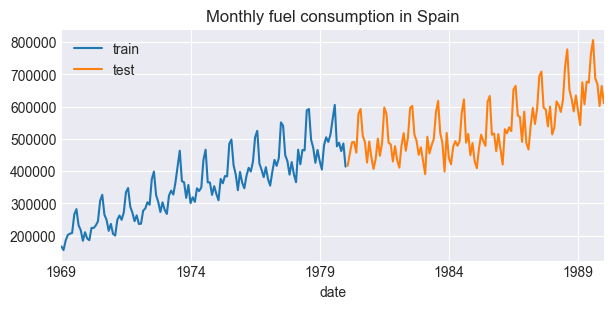## Exploratory Analysis: Stationarity and Differentiation¶

Embarking on the journey to create an ARIMA model requires a comprehensive exploratory analysis. This critical step serves as a compass, guiding the analyst toward a deep understanding of the intrinsic dynamics of the data. Before fitting an ARIMA model to time series data, it is important to conduct an exploratory analysis to determine, at least, the following:

• Stationarity: Stationarity means that the statistical properties (mean, variance...) remain constant over time, so time series with trends or seasonality are not stationary. Since ARIMA assumes the stationarity of the data, it is essential to subject the data to rigorous tests, such as the Augmented Dickey-Fuller test, to assess stationarity. If non-stationarity is found, the series should be differenced until stationarity is achieved. This analysis helps to determine the optimal value of the parameter $d$.

• Autocorrelation analysis: Plot the autocorrelation and partial autocorrelation functions (ACF and PACF) to identify potential lag relationships between data points. This visual analysis provides insight into determining appropriate autoregressive (AR) and moving average (MA) terms ($p$ and $q$) for the ARIMA model.

• Seasonal decomposition: In cases where seasonality is suspected, decomposing the series into trend, seasonal, and residual components using techniques such as moving averages or seasonal time series decomposition (STL) can reveal hidden patterns and help identify seasonality. This analysis helps to determine the optimal values of the parameters $P$, $D$, $Q$ and $m$.

These exploratory analyses establish the foundation for constructing an effective ARIMA model that captures the fundamental patterns and associations within the data.

### Stationarity¶

There are several methods to assess whether a given time series is stationary or non-stationary:

• Visual inspection of the time series: By visually inspecting the time series plot, it becomes possible to identify the presence of a noticeable trend or seasonality. If such patterns are apparent, it is probable that the series is non-stationary.

• Summary statistics: Calculate summary statistics, such as the mean and variance, for various segments of the series. If significant differences exist, the series is not stationary.

• Statistical tests: Apply statistical tests such as the Augmented Dickey-Fuller test or the Kwiatkowski-Phillips-Schmidt-Shin (KPSS) test.

The previous plot shows a clear positive trend in the series, indicating a steady increase over time. Consequently, the mean of the series increases over time, confirming its non-stationarity.

Differencing is one of the easiest techniques to detrend a time series. A new series is generated where the value at the current time step is calculated as the difference between the original observation and the observation at the previous time step, i.e. the difference between consecutive values. Mathematically, the first difference is calculated as:

$$\Delta X_t = X_t - X_{t-1}$$

Where $X_t$ is the value at time $t$ and $X_{t-1}$ is the value at time $t-1$. This is known as first order differentiation. This process can be repeated if necessary until the desired stationarity is reached.

In the following sections, the original time series is subjected to both first and second-order differencing and statistical tests are applied to determine whether stationarity is achieved.

Augmented Dickey-Fuller test

The Augmented Dickey-Fuller test takes as its null hypothesis that the time series has a unit root - a characteristic of non-stationary time series. Conversely, the alternative hypothesis (under which the null hypothesis is rejected) is that the series is stationary.

• Null Hypothesis (HO): The series is not stationary or has a unit root.

• Alternative hypothesis (HA): The series is stationary with no unit root.

Since the null hypothesis assumes the presence of a unit root, the p-value obtained should be less than a specified significance level, often set at 0.05, to reject this hypothesis. This result indicates the stationarity of the series. The adfuller() function within the Statsmodels library is a handy tool for implementing the ADF test. Its output includes four values: the p-value, the value of the test statistic, the number of lags included in the test, and critical value thresholds for three different levels of significance.

Kwiatkowski-Phillips-Schmidt-Shin test (KPSS)

The KPSS test checks if a time series is stationary around a mean or linear trend. In this test, the null hypothesis is that the data are stationary, and we look for evidence that the null hypothesis is false. Consequently, small p-values (e.g., less than 0.05) rejects the null hypothesis and suggest that differencing is required. Statsmodels library provides an implementation of the KPSS test via the kpss() function.

Note

While both tests are used to check stationarity,
• The KPSS test focuses on the presence of trends, and a low p-value indicates non-stationarity due to a trend.
• The ADF test focuses on the presence of a unit root, and a low p-value indicates that the time series does not have a unit root, suggesting it might be stationary.
It's common to use both tests together to get a more comprehensive understanding of the stationarity properties of a time series.
In :
# Test stationarity
# ==============================================================================
warnings.filterwarnings("ignore")

data_diff_1 = data_train.diff().dropna()
data_diff_2 = data_diff_1.diff().dropna()

print('Test stationarity for original series')
print('-------------------------------------')
kpss_result = kpss(data)
print(f'KPSS Statistic: {kpss_result}, p-value: {kpss_result}')

print('\nTest stationarity for differenced series (order=1)')
print('--------------------------------------------------')
kpss_result = kpss(data.diff().dropna())
print(f'KPSS Statistic: {kpss_result}, p-value: {kpss_result}')

print('\nTest stationarity for differenced series (order=2)')
print('--------------------------------------------------')
kpss_result = kpss(data.diff().diff().dropna())
print(f'KPSS Statistic: {kpss_result}, p-value: {kpss_result}')

warnings.filterwarnings("default")

# Plot series
# ==============================================================================
fig, axs = plt.subplots(nrows=3, ncols=1, figsize=(7, 5), sharex=True)
data.plot(ax=axs, title='Original time series')
data_diff_1.plot(ax=axs, title='Differenced order 1')
data_diff_2.plot(ax=axs, title='Differenced order 2');

Test stationarity for original series
-------------------------------------
KPSS Statistic: 2.2096370946978383, p-value: 0.01

Test stationarity for differenced series (order=1)
--------------------------------------------------
KPSS Statistic: 0.3132711623572787, p-value: 0.1

Test stationarity for differenced series (order=2)
--------------------------------------------------
KPSS Statistic: 0.08065668267482227, p-value: 0.1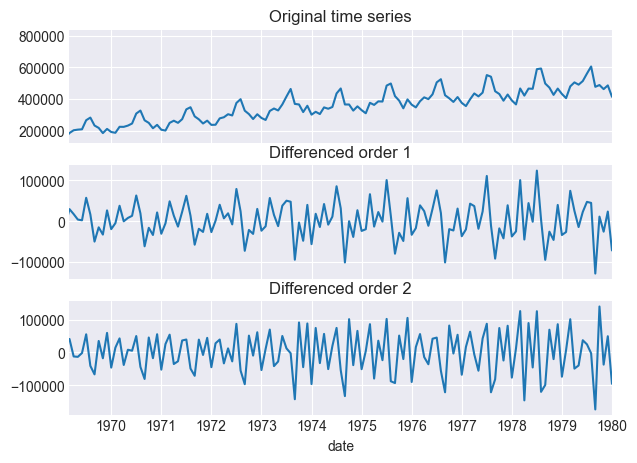After checking the first and second-order differences, the p-value indicates a statistically significant decrease below the widely-recognized and accepted threshold of 0.05 for order=1. Therefore, the most appropriate selection for the ARIMA parameter $d$ is 1.

### Autocorrelation Analysis¶

Plotting the autocorrelation function (ACF) and partial autocorrelation function (PACF) of the time series can provide insight into the appropriate values for $p$ and $q$. The ACF helps in identifying the value of $q$ (lag in the moving average part), while the PACF assists in identifying the value of $p$ (lag in the autoregressive part).

Warning

If the stationarity analysis indicates that differencing is required, subsequent analyses should be conducted using the differenced series, as this will align with the manner in which the ARIMA model interprets the series.

Autocorrelation Function (ACF)

The ACF calculates the correlation between a time series and its lagged values. Within the context of ARIMA modeling, a sharp drop-off in the ACF after a few lags indicates that the data have a finite autoregressive order. The lag at which the ACF drops off provides an estimation of the value of $q$. If the ACF displays a sinusoidal or damped sinusoidal pattern, it suggests seasonality is present and requires consideration of seasonal orders in addition to non-seasonal orders.

Partial Autocorrelation Function (PACF)

The PACF measures the correlation between a lagged value and the current value of the time series, while accounting for the effect of the intermediate lags. In the context of ARIMA modeling, if the PACF sharply cuts off after a certain lag, while the remaining values are within the confidence interval, it suggests an AR model of that order. The lag, at which the PACF cuts off, gives an idea of the value of $p$.

Note

Some rules of thumb are:
• Take the order of AR term p to be equal to as many lags that crosses the significance limit in the PACF plot.

• Take the order of MA term q to be equal to as many lags that crosses the significance limit in the ACF plot.

• If the ACF cuts off at lag q and the PACF cuts off at lag p, one could start with an ARIMA(p, d, q) model.

• If only the PACF stops after lag p, one could start with an AR(p) model.

• If only the ACF stops after lag q, one could start with an MA(q) model.

These guidelines provide a useful starting point when selecting the orders of an ARIMA model and can be adjusted according to the specific characteristics of the data in question.
In :
# Autocorrelation plot for original and differenced series
# ==============================================================================
fig, axs = plt.subplots(nrows=2, ncols=1, figsize=(7, 4), sharex=True)
plot_acf(data, ax=axs, lags=50, alpha=0.05)
axs.set_title('Autocorrelation original series')
plot_acf(data_diff_1, ax=axs, lags=50, alpha=0.05)
axs.set_title('Autocorrelation differenced series (order=1)');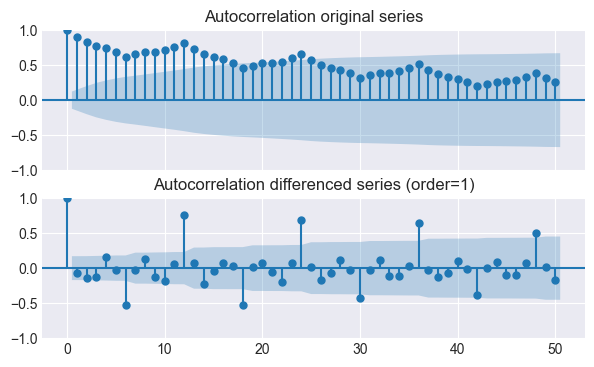In :
# Partial autocorrelation plot for original and differenced series
# ==============================================================================
fig, axs = plt.subplots(nrows=2, ncols=1, figsize=(7, 3), sharex=True)
plot_pacf(data, ax=axs, lags=50, alpha=0.05)
axs.set_title('Partial autocorrelation original series')
plot_pacf(data_diff_1, ax=axs, lags=50, alpha=0.05)
axs.set_title('Partial autocorrelation differenced series (order=1)');
plt.tight_layout();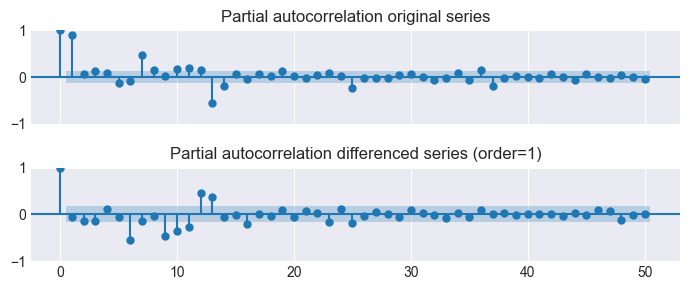Based on the autocorrelation function, the optimal value for parameter $p$ is 0. However, we will assign a value of 1 to provide an autoregressive component to the model. Regarding the $q$ component, the partial autocorrelation function suggests a value of 1.

### Time serie descomposition¶

Time series decomposition involves breaking down the original time series into its underlying components, namely trend, seasonality, and residual (error) components. The decomposition can be either additive or multiplicative. Including time series decomposition along with ACF and PACF analysis provides a comprehensive approach to understanding the underlying structure of the data and choose appropriate ARIMA parameters.

In :
# Time series descoposition of original versus differenced series
# ==============================================================================

fig, axs = plt.subplots(nrows=4, ncols=2, figsize=(9, 6), sharex=True)

res_decompose.observed.plot(ax=axs[0, 0])
axs[0, 0].set_title('Original series')
res_decompose.trend.plot(ax=axs[1, 0])
axs[1, 0].set_title('Trend')
res_decompose.seasonal.plot(ax=axs[2, 0])
axs[2, 0].set_title('Seasonal')
res_decompose.resid.plot(ax=axs[3, 0])
axs[3, 0].set_title('Residuals')
res_descompose_diff_2.observed.plot(ax=axs[0, 1])
axs[0, 1].set_title('Differenced series (order=1)')
res_descompose_diff_2.trend.plot(ax=axs[1, 1])
axs[1, 1].set_title('Trend')
res_descompose_diff_2.seasonal.plot(ax=axs[2, 1])
axs[2, 1].set_title('Seasonal')
res_descompose_diff_2.resid.plot(ax=axs[3, 1])
axs[3, 1].set_title('Residuals')
fig.suptitle('Time serie decomposition original series versus differenced series', fontsize=14)
fig.tight_layout();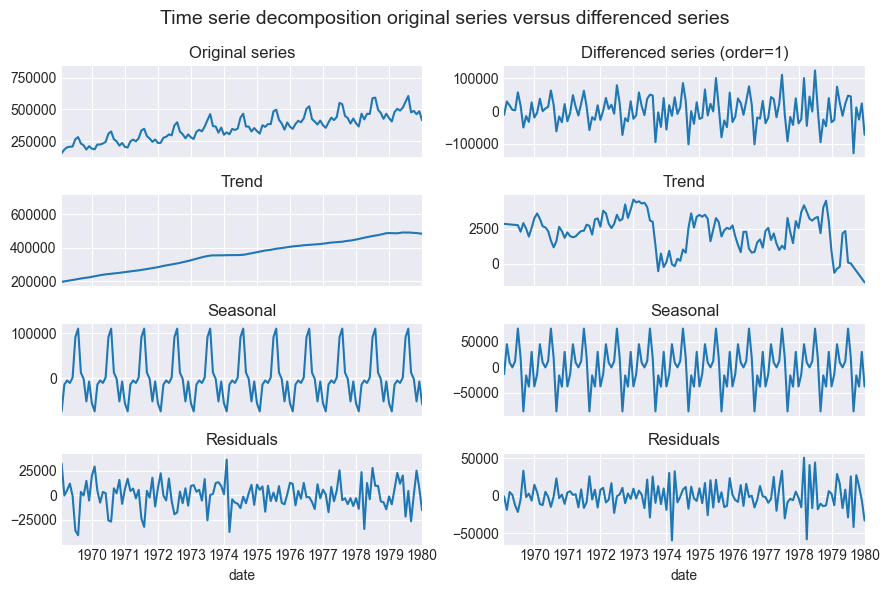The recurring pattern every 12 months suggests an annual seasonality, likely influenced by holiday factors. The ACF plot further supports the presence of seasonality, as significant peaks occur at lags corresponding to the 12-month intervals, confirming the idea of recurring patterns.

### Conclusions¶

Based on the results of the exploratory analysis, utilizing a combination of first-order differencing and seasonal differencing may be the most appropriate approach. First-order differencing is effective in capturing transitions between observations and highlighting short-term fluctuations. Concurrently, seasonal differencing, which covers a period of 12 months and represents the shift from one year to the next, effectively captures the inherent cyclic patterns in the data. This approach allows us to achieve the necessary stationarity for the following ARIMA modeling process.

In :
# First order differentiation combined with seasonal differentiation
# ==============================================================================
data_diff_1_12 = data_train.diff().diff(12).dropna()

warnings.filterwarnings("ignore")
kpss_result = kpss(data_diff_1_12)
print(f'KPSS Statistic: {kpss_result}, p-value: {kpss_result}')
warnings.filterwarnings("default")

ADF Statistic: -4.387457230769961, p-value: 0.00031237732711268733
KPSS Statistic: 0.06291573421251054, p-value: 0.1


Warning

Exploratory data analysis is an evolving and iterative process in which the insights gained have the potential to change as the process progresses. Remember that all previous plots only provide initial guidance, and the optimal values of $p$, $q$, and $d$ should be selected based on a combination of these plots, statistical criteria such as AIC and BIC, and a time series validation such as backtesting.

## ARIMA-SARIMAX model¶

The following section focus on how to train an ARIMA model and forecast future values with each of the three libraries.

### Statsmodels¶

In statsmodels, a distinction is made between the process of defining a model and training it. This approach may be familiar to R programming language users, but it may seem somewhat unconventional to those accustomed to libraries like scikit-learn or XGBoost in the Python ecosystem.

The process begins with the model definition, which includes configurable parameters and the training dataset. When the fit method is invoked, instead of modifying the model object, as is typical in Python libraries, statsmodels creates a new SARIMAXResults object. This object not only encapsulates essential details like residuals and learned parameters, but also provides the necessary tools to generate predictions.

In :
# ARIMA model with statsmodels.Sarimax
# ==============================================================================
warnings.filterwarnings("ignore", category=UserWarning, message='Non-invertible|Non-stationary')
model = SARIMAX(endog = data_train, order = (1, 1, 1), seasonal_order = (1, 1, 1, 12))
model_res = model.fit(disp=0)
warnings.filterwarnings("default")

model_res.summary()

c:\anaconda\envs\skforecast_10_py11\Lib\site-packages\scipy\stats\_stats_py.py:1069: DeprecationWarning: Conversion of an array with ndim > 0 to a scalar is deprecated, and will error in future. Ensure you extract a single element from your array before performing this operation. (Deprecated NumPy 1.25.)
else dtype(mean))

Out:
Dep. Variable: No. Observations: litters 133 SARIMAX(1, 1, 1)x(1, 1, 1, 12) -1356.051 Fri, 15 Sep 2023 2722.103 15:41:43 2736.040 01-01-1969 2727.763 - 01-01-1980 opg
coef std err z P>|z| [0.025 0.975] -0.4972 0.134 -3.707 0.000 -0.760 -0.234 -0.0096 0.146 -0.066 0.947 -0.295 0.276 0.0465 0.162 0.288 0.774 -0.270 0.364 -0.3740 0.203 -1.847 0.065 -0.771 0.023 3.291e+08 1.06e-09 3.1e+17 0.000 3.29e+08 3.29e+08
 Ljung-Box (L1) (Q): Jarque-Bera (JB): 5.13 18.12 0.02 0 1.26 -0.42 0.46 4.71

Warnings:
 Covariance matrix calculated using the outer product of gradients (complex-step).
 Covariance matrix is singular or near-singular, with condition number 1.36e+33. Standard errors may be unstable.

The model summary shows a lot of information about the fitting process:

• Model Fit Statistics: This part includes several statistics that help you evaluate how well the model fits the data:

• Log-Likelihood: A measure of how well the model explains the observed data. When fitting an ARIMA model, negative log-likelihood values will be encounter, with more negative values indicating a poorer fit to the data, and values closer to zero indicating a better fit.

• AIC (Akaike Information Criterion): A goodness-of-fit metric that balances the fit of the model with its complexity. Lower AIC values are preferred.

• BIC (Bayesian Information Criterion): Similar to AIC, but penalizes model complexity more. As with AIC, lower BIC values are better.

• HQIC (Hannan-Quinn Information Criterion): Another model selection criterion, similar to AIC and BIC.

• Coefficients: This table lists the estimated coefficients for the parameters of the model. It includes both autoregressive (AR) and moving average (MA) parameters, as well as any exogenous variables if they are included in the model. It also includes the standard errors associated with the estimated coefficients to indicate the uncertainty in the parameter estimates, their P-values, which are used to assess the significance of each coefficient, and the 95% confidence interval.

• Model diagnostics: This section provides information about the residuals (the differences between the observed values (training values) and their predicted values from the model):

• Ljung-Box test: A test for autocorrelation in the residuals.

• Jarque-Bera test: A test of the normality of the residuals.

• Skewness and kurtosis: Measures of the shape of the distribution of the residuals.

In :
# Prediction
# ==============================================================================
predictions_statsmodels = model_res.get_forecast(steps=len(data_test)).predicted_mean
predictions_statsmodels.name = 'predictions_statsmodels'

1980-02-01    407504.056972
1980-03-01    473997.245818
1980-04-01    489983.091498
1980-05-01    485517.462878
Freq: MS, Name: predictions_statsmodels, dtype: float64

### Skforecast¶

Skforecast wraps the statsmodels.SARIMAX model and adapts it to the scikit-learn API.

In :
# ARIMA model with skforecast.Sarimax
# ==============================================================================
warnings.filterwarnings("ignore", category=UserWarning, message='Non-invertible|Non-stationary')

model = Sarimax(order=(1, 1, 1), seasonal_order=(1, 1, 1, 12))
model.fit(y=data_train)
model.summary()

warnings.filterwarnings("default")

c:\anaconda\envs\skforecast_10_py11\Lib\site-packages\scipy\stats\_stats_py.py:1069: DeprecationWarning: Conversion of an array with ndim > 0 to a scalar is deprecated, and will error in future. Ensure you extract a single element from your array before performing this operation. (Deprecated NumPy 1.25.)
else dtype(mean))

In :
# Prediction
# ==============================================================================
predictions_skforecast = model.predict(steps=len(data_test))
predictions_skforecast.columns = ['skforecast']

skforecast
1980-02-01 407504.056972
1980-03-01 473997.245818
1980-04-01 489983.091498
1980-05-01 485517.462878

Note

Since skforecast Sarimax, is a wrapper of statsmodels SARIMAX, the results are the same.

## pdmarima¶

In :
# ARIMA model with pdmarima.Sarimax
# ==============================================================================
model = ARIMA(order=(1, 1, 1), seasonal_order=(1, 1, 1, 12))
model.fit(y=data_train)
model.summary()

c:\anaconda\envs\skforecast_10_py11\Lib\site-packages\scipy\stats\_stats_py.py:1069: DeprecationWarning: Conversion of an array with ndim > 0 to a scalar is deprecated, and will error in future. Ensure you extract a single element from your array before performing this operation. (Deprecated NumPy 1.25.)
else dtype(mean))

Out:
Dep. Variable: No. Observations: y 133 SARIMAX(1, 1, 1)x(1, 1, 1, 12) -1355.749 Fri, 15 Sep 2023 2723.498 15:41:45 2740.223 01-01-1969 2730.290 - 01-01-1980 opg
coef std err z P>|z| [0.025 0.975] -474.5820 1101.722 -0.431 0.667 -2633.917 1684.753 -0.4896 0.138 -3.554 0.000 -0.760 -0.220 -0.0211 0.151 -0.139 0.889 -0.317 0.275 0.0545 0.164 0.331 0.740 -0.268 0.377 -0.3841 0.204 -1.884 0.060 -0.784 0.015 3.289e+08 0.002 1.84e+11 0.000 3.29e+08 3.29e+08
 Ljung-Box (L1) (Q): Jarque-Bera (JB): 4.9 18.55 0.03 0 1.27 -0.43 0.46 4.73

Warnings:
 Covariance matrix calculated using the outer product of gradients (complex-step).
 Covariance matrix is singular or near-singular, with condition number 4.08e+27. Standard errors may be unstable.
In :
# Prediction
# ==============================================================================
predictions_pdmarima = model.predict(len(data_test))
predictions_pdmarima.name = 'predictions_pdmarima'

1980-02-01    406998.311392
1980-03-01    472944.444486
1980-04-01    488389.125309
1980-05-01    483432.075700
Freq: MS, Name: predictions_pdmarima, dtype: float64
In :
# Plot predictions
# ==============================================================================
fig, ax = plt.subplots(figsize=(7, 3))
data_train.plot(ax=ax, label='train')
data_test.plot(ax=ax, label='test')
predictions_statsmodels.plot(ax=ax, label='statsmodels')
predictions_skforecast.plot(ax=ax, label='skforecast')
predictions_pdmarima.plot(ax=ax, label='pmdarima')
ax.set_title('Predictions with ARIMA models')
ax.legend();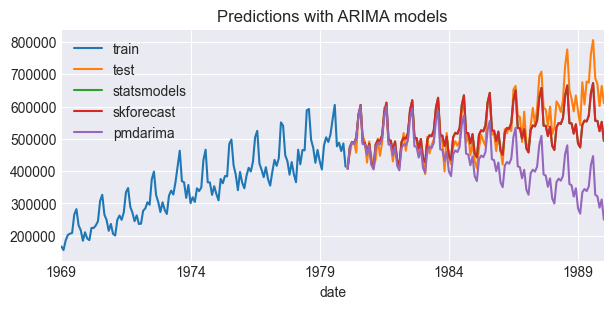Warning

While pdmarima works as a wrapper for statmodels SARIMAX, it's worth noting that the results diverge. At the time of writing, the authors are investigating the causes of the lack of reproducibility.

## ForecasterSarimax¶

The ForecasterSarimax class allows training and validation of ARIMA and SARIMAX models using the skforecast API. ForecasterSarimax is compatible with two ARIMA-SARIMAX implementations:

• ARIMA from pmdarima: a wrapper for statsmodels SARIMAX that follows the scikit-learn API.

• Sarimax from skforecast: a novel wrapper for statsmodels SARIMAX that also follows the sklearn API. This implementation is very similar to pmdarima, but has been streamlined to include only the essential elements for skforecast, resulting in significant speed improvements.

Since ForecasterSarimax follows the same API as the other Forecasters available in the library, it is very easy to make a fair and robust comparison of an ARIMA-SARIMAX performance against other machine learning models such as Random Forest or Gradient Boosting.

### Train - Predict¶

The train-prediction process follows an API similar to that of scikit-learn. ForecasterSarimax user guide.

In :
# ARIMA model with ForecasterSarimax and skforecast Sarimax
# ==============================================================================
forecaster = ForecasterSarimax(
regressor=Sarimax(order=(1, 1, 1), seasonal_order=(1, 1, 1, 12))
)
forecaster.fit(y=data_train, suppress_warnings=True)

# Prediction
predictions = forecaster.predict(steps=len(data_test))

Out:
1980-02-01    407504.056972
1980-03-01    473997.245818
1980-04-01    489983.091498
1980-05-01    485517.462878
Freq: MS, Name: pred, dtype: float64

### Backtesting¶

The following example shows the application of backtesting in assessing the performance of the SARIMAX model when generating forecasts for the upcoming 12 months on an annual schedule. In this context, a forecast is generated at the end of each December, predicting values for the subsequent 12 months.

Tip

If suppress_warnings_fit=True warnings generated during fitting will be ignored.
In :
# Backtest forecaster
# ==============================================================================
forecaster = ForecasterSarimax(
regressor=Sarimax(
order=(1, 1, 1),
seasonal_order=(1, 1, 1, 12),
maxiter=200
)
)

metric, predictions = backtesting_sarimax(
forecaster            = forecaster,
y                     = data,
initial_train_size    = len(data_train),
fixed_train_size      = False,
steps                 = 12,
metric                = 'mean_absolute_error',
refit                 = True,
n_jobs                = "auto",
suppress_warnings_fit = True,
verbose               = True,
show_progress         = True
)

print(f"Metric (mean_absolute_error): {metric}")

Information of backtesting process
----------------------------------
Number of observations used for initial training: 133
Number of observations used for backtesting: 120
Number of folds: 10
Number of steps per fold: 12
Number of steps to exclude from the end of each train set before test (gap): 0

Fold: 0
Training:   1969-01-01 00:00:00 -- 1980-01-01 00:00:00  (n=133)
Validation: 1980-02-01 00:00:00 -- 1981-01-01 00:00:00  (n=12)
Fold: 1
Training:   1969-01-01 00:00:00 -- 1981-01-01 00:00:00  (n=145)
Validation: 1981-02-01 00:00:00 -- 1982-01-01 00:00:00  (n=12)
Fold: 2
Training:   1969-01-01 00:00:00 -- 1982-01-01 00:00:00  (n=157)
Validation: 1982-02-01 00:00:00 -- 1983-01-01 00:00:00  (n=12)
Fold: 3
Training:   1969-01-01 00:00:00 -- 1983-01-01 00:00:00  (n=169)
Validation: 1983-02-01 00:00:00 -- 1984-01-01 00:00:00  (n=12)
Fold: 4
Training:   1969-01-01 00:00:00 -- 1984-01-01 00:00:00  (n=181)
Validation: 1984-02-01 00:00:00 -- 1985-01-01 00:00:00  (n=12)
Fold: 5
Training:   1969-01-01 00:00:00 -- 1985-01-01 00:00:00  (n=193)
Validation: 1985-02-01 00:00:00 -- 1986-01-01 00:00:00  (n=12)
Fold: 6
Training:   1969-01-01 00:00:00 -- 1986-01-01 00:00:00  (n=205)
Validation: 1986-02-01 00:00:00 -- 1987-01-01 00:00:00  (n=12)
Fold: 7
Training:   1969-01-01 00:00:00 -- 1987-01-01 00:00:00  (n=217)
Validation: 1987-02-01 00:00:00 -- 1988-01-01 00:00:00  (n=12)
Fold: 8
Training:   1969-01-01 00:00:00 -- 1988-01-01 00:00:00  (n=229)
Validation: 1988-02-01 00:00:00 -- 1989-01-01 00:00:00  (n=12)
Fold: 9
Training:   1969-01-01 00:00:00 -- 1989-01-01 00:00:00  (n=241)
Validation: 1989-02-01 00:00:00 -- 1990-01-01 00:00:00  (n=12)


Metric (mean_absolute_error): 19611.23634640705

pred
1980-02-01 407504.056972
1980-03-01 473997.245818
1980-04-01 489983.091498
1980-05-01 485517.462878
In :
# Plot backtest predictions
# ==============================================================================
fig, ax = plt.subplots(figsize=(6, 3))
data.loc[end_train:].plot(ax=ax, label='test')
predictions.plot(ax=ax)
ax.set_title('Backtest predictions with SARIMAX model')
ax.legend();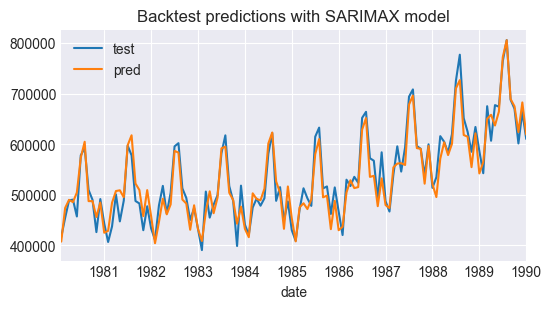### Model tuning¶

The exploratory analysis has successfully narrowed down the search space for the optimal hyperparameters of the model. However, to definitively determine the most appropriate values, the use of strategic search methods is essential. Among these methods, two widely used approaches are:

• Statistical Criteria: Information criterion metrics, such as Akaike's Information Criterion (AIC) or Bayesian Information Criterion (BIC), use different penalties on the maximum likelihood (log-likelihood) estimate of the model as a measure of fit. The advantage of using such criteria is that they are computed only on the training data, eliminating the need for predictions on new data. As a result, the optimization process is greatly accelerated. The well-known Auto Arima algorithm uses this approach.

• Validation Techniques: The use of validation techniques, especially backtesting, is another effective strategy. Backtesting involves evaluating the performance of the model using historical data to simulate real-world conditions. This helps to validate the effectiveness of the hyperparameters under different scenarios, providing a practical assessment of their viability.

In the first approach, calculations are based solely on training data, eliminating the need for predictions on new data. This makes the optimization process very fast. However, it is important to note that information criteria metrics only measure the relative quality of models. This means that all tested models could still be poor fits. Therefore, the final selected model must undergo a backtesting phase. This phase calculates a metric (such as MAE, MSE, MAPE, etc.) that validates its performance on a meaningful scale.

On the other hand, the second approach - validation techniques - tends to be more time-consuming, since the model must be trained and then evaluated on new data. However, the results generated are often more robust, and the metrics derived can provide deeper insights.

Tip

In summary, while the statistical criteria approach offers speed and efficiency, validation techniques provide a more comprehensive and insightful evaluation, albeit at a slower pace due to their reliance on new data for testing. Fortunately, for sufficiently large data sets, they all lead to the same model.

Warning

When evaluating ARIMA-SARIMAX models, it is important to note that AIC assumes that all models are trained on the same data. Thus, using AIC to decide between different orders of differencing is technically invalid, since one data point is lost with each order of differencing. Therefore, the Auto Arima algorithm uses a unit root test to select the order of differencing, and only uses the AIC to select the order of the AR and MA components.

Note

For a detailed explanation of Akaike's Information Criterion (AIC) see Rob J Hyndman's blog and AIC Myths and Misunderstandings by Anderson and Burnham.

It is crucial to conduct hyperparameter optimization using a validation dataset, rather than the test dataset, to ensure a accurate evaluation of model performance.

In :
# Train-validation-test data
# ======================================================================================
end_train = '1976-01-01 23:59:59'
end_val = '1984-01-01 23:59:59'
print(
f"Train dates      : {data.index.min()} --- {data.loc[:end_train].index.max()}  "
f"(n={len(data.loc[:end_train])})"
)
print(
f"Validation dates : {data.loc[end_train:].index.min()} --- {data.loc[:end_val].index.max()}  "
f"(n={len(data.loc[end_train:end_val])})"
)
print(
f"Test dates       : {data.loc[end_val:].index.min()} --- {data.index.max()}  "
f"(n={len(data.loc[end_val:])})"
)

# Plot
# ======================================================================================
fig, ax = plt.subplots(figsize=(7, 3))
data.loc[:end_train].plot(ax=ax, label='train')
data.loc[end_train:end_val].plot(ax=ax, label='validation')
data.loc[end_val:].plot(ax=ax, label='test')
ax.set_title('Monthly fuel consumption in Spain')
ax.legend();

Train dates      : 1969-01-01 00:00:00 --- 1976-01-01 00:00:00  (n=85)
Validation dates : 1976-02-01 00:00:00 --- 1984-01-01 00:00:00  (n=96)
Test dates       : 1984-02-01 00:00:00 --- 1990-01-01 00:00:00  (n=72)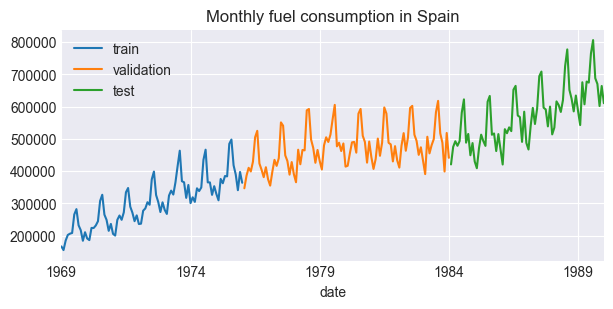In :
# Grid search based on backtesting
# ==============================================================================
forecaster = ForecasterSarimax(
regressor=Sarimax(order=(1, 1, 1), maxiter=500), # Placeholder replaced in the grid search
)

param_grid = {
'order': [(0, 1, 0), (0, 1, 1), (1, 1, 0), (1, 1, 1), (2, 1, <`